Related Articles

# Implement dynamic deque using templates class and a circular array

• Difficulty Level : Medium
• Last Updated : 21 May, 2021

The task is to implement a dynamic Deque using templates class and a circular array, having the following functionalities:

• front(): Get the front item from the deque.
• back(): Get the last item from the deque.
• push_back(X): Push X at the end of the deque.
• push_front(X): Push X at the start of the deque.
• pop_front(): Delete an element from the start of the deque.
• pop_back(): Delete an element from the end of the deque
• empty(): Checks whether deque is empty or not
• capacity(): Current maximum number of elements deque can store
• size(): Number of elements in the deque

Below is the step by step illustration:

• Initially, the deque is empty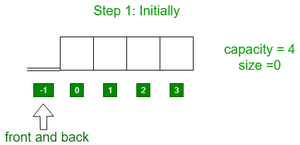• Insert 1 to the back of deque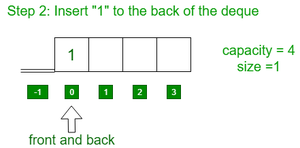• Insert elements 2, 3 to the back of deque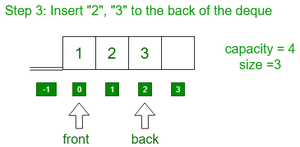• Insert 4 to the front of deque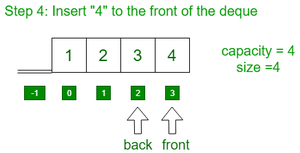• Insert 5 to the back of deque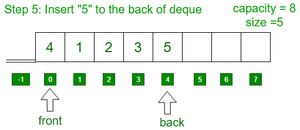• Pop 2 elements from front and 2 elements from the back of deque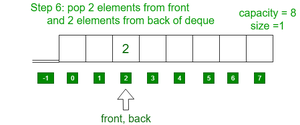• Pop 1 element from the front of deque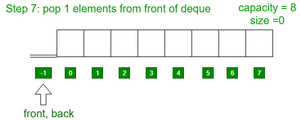Approach: The idea is to double the size of the array used every time the capacity of the array gets full and copy the elements of the previous array into the new array. Follow the steps below to solve the problem:

• Initialize 4 variables say frontIndex, backIndex, sizeVar, and capacityVar, and an array say arr[] to implement the deque.
• Define a function say capacity() to find the size of the current array used and return the value of the variable, capacityVar.
• Define a function say size() to find the count of elements in the deque and return the value of the variable, sizeVar.
• Define a function say full() to find if the deque is full or not and return true if sizeVar is equal to capacityVar. Otherwise, return false.
• Define a function say empty() to find if the deque is empty or not and return true if frontIndex and backIndex are equal to -1. Otherwise, return false.
• Define a function say Front() to print the front element of the deque. If deque is not empty(), print the element of arr[frontIndex].
• Define a function say Back() to print the last element of the deque. If deque is not empty(), print the element of arr[BackIndex].
• Define a function say push_back(X) to insert an element at the end of the deque:
• If the deque is full, then double the size of the current array and copy the elements of the previous array into the new array.
• If deque is empty(), then assign frontIndex = backIndex = 0 and then assign X to both arr[frontIndex] and arr[backIndex] and then increment sizeVar by one.
• Else, update backIndex as backIndex = (backIndex+1) %capacityVar and then assign X to arr[backIndex] and increment sizeVar by one.
• Define a function say push_front(X) to insert an element at the start of the deque:
• If the deque is full, then double the size of the current array and copy the elements of the previous array into the new array.
• If deque is empty(), then assign frontIndex = backIndex = 0 and then assign X to both arr[frontIndex] and arr[backIndex] and then increment sizeVar by one.
• Else, update frontIndex as frontIndex = (frontIndex-1 + capacityVar)%capacityVar and then assign X to arr[frontIndex] and increment sizeVar by one.
• Define a function say pop_front() to delete an element at the front of the deque:
• If the deque is empty, print “Underflow”.
• Else if sizeVar is equal to 1 then assign -1 to frontIndex and backIndex both and then decrement sizeVar by one.
• Else, Update frontIndex as frontIndex = (frontIndex+1)%capacityVar and decrement sizeVar by one.
• Define a function say pop_back() to delete an element at the front of the deque:
• If the deque is empty, print “Underflow”.
• Else if sizeVar is equal to 1 then assign -1 to frontIndex and backIndex both and then decrement sizeVar by one.
• Else, Update backIndex as backIndex = (backndex-1 + capacityVar) %capacityVar and decrement sizeVar by one.

Below is the implementation of the above approach:

## C++

 `// C++ program for the above approach``#include ``using` `namespace` `std;` `// Class definition of the deque``template` `<``class` `X>``class` `Deque {` `private``:``    ``// Stores the frontIndex``    ``int` `frontIndex;` `    ``// Stores the backIndex``    ``int` `backIndex;` `    ``// Stores the array``    ``X* arr;` `    ``// Stores the size of deque``    ``int` `sizeVar;` `    ``// Stores the size of array``    ``int` `capacityVar = 4;` `public``:``    ``// Deque class constructor``    ``Deque()``    ``{``        ``arr = ``new` `X[capacityVar];``        ``frontIndex = backIndex = -1;``        ``sizeVar = 0;``    ``}` `    ``// Function methods``    ``bool` `empty();``    ``bool` `full();``    ``void` `push_back(X x);``    ``void` `push_front(X x);``    ``void` `pop_front();``    ``void` `pop_back();``    ``X front();``    ``X back();``    ``int` `capacity();``    ``int` `size();``};` `// Function to find the capacity of the deque``template` `<``class` `X>``int` `Deque::capacity()``{``    ``return` `capacityVar;``}` `// Function to find the number of elements``// present in deque``template` `<``class` `X>``int` `Deque::size() { ``return` `sizeVar; }` `// Function to check if deque is empty or not``template` `<``class` `X>``bool` `Deque::empty()``{``    ``if` `(frontIndex == -1 && backIndex == -1)``        ``return` `true``;``    ``else``        ``return` `false``;``}` `// Function to check if deque is full or not``template` `<``class` `X>``bool` `Deque::full()``{``    ``if` `(sizeVar == capacityVar)``        ``return` `true``;``    ``else``        ``return` `false``;``}` `// Function to find the front element of the deque``template` `<``class` `X>``X Deque::front()``{``    ``// If deque is empty``    ``if` `(empty()) {``        ``cout << ``"Deque underflow"` `<< endl;``        ``abort``();``    ``}``    ``return` `arr[frontIndex];``}` `// Function to find the last element of the deque``template` `<``class` `X>``X Deque::back()``{``    ``// If deque is empty``    ``if` `(empty()) {``        ``cout << ``"Deque underflow"` `<< endl;``        ``abort``();``    ``}``    ``return` `arr[backIndex];``}` `// Function to insert the element``// to the back of the deque``template` `<``class` `X>``void` `Deque::push_back(X x)``{``    ``if` `(full()) {` `        ``// If the deque is full, then``        ``// double the capacity``        ``capacityVar = capacityVar * 2;` `        ``// Initialize new array of``        ``// double size``        ``X* temp = ``new` `X[capacityVar];` `        ``// Copy the elements of the``        ``// previous array``        ``int` `i = frontIndex;``        ``int` `j = 0;``        ``while` `(i != backIndex) {``            ``temp[j] = arr[i];``            ``i = (i + 1) % sizeVar;``            ``j++;``        ``}``        ``temp[j] = arr[i];` `        ``frontIndex = 0;``        ``backIndex = sizeVar - 1;` `        ``// Deallocate the memory``        ``// of previous array``        ``delete``[] arr;``        ``arr = temp;``    ``}` `    ``// If size is zero``    ``if` `(empty()) {``        ``frontIndex = backIndex = 0;``        ``arr[backIndex] = x;``        ``sizeVar++;``        ``return``;``    ``}` `    ``// Increment back index cyclically``    ``backIndex = (backIndex + 1) % capacityVar;``    ``arr[backIndex] = x;``    ``sizeVar++;``    ``return``;``}` `// Function to insert the element``// to the front of the deque``template` `<``class` `X>``void` `Deque::push_front(X x)``{``    ``if` `(full()) {` `        ``// If the deque is full, then``        ``// double the capacity``        ``capacityVar = capacityVar * 2;` `        ``// Initialize new array of``        ``// double size``        ``X* temp = ``new` `X[capacityVar];` `        ``// Copy the elements of the``        ``// previous array``        ``int` `i = frontIndex;``        ``int` `j = 0;``        ``while` `(i != backIndex) {``            ``temp[j] = arr[i];``            ``i = (i + 1) % sizeVar;``            ``j++;``        ``}``        ``temp[j] = arr[i];` `        ``frontIndex = 0;``        ``backIndex = sizeVar - 1;` `        ``// Deallocate the memory``        ``// of previous array``        ``delete``[] arr;``        ``arr = temp;``    ``}` `    ``// If size is zero``    ``if` `(empty()) {``        ``frontIndex = backIndex = 0;``        ``arr[backIndex] = x;``        ``sizeVar++;``        ``return``;``    ``}` `    ``// Decrement front index cyclically``    ``frontIndex``        ``= (frontIndex - 1 + capacityVar) % capacityVar;``    ``arr[frontIndex] = x;``    ``sizeVar++;``    ``return``;``}` `// Function to delete the element``// from the front of the deque``template` `<``class` `X>``void` `Deque::pop_front()``{``    ``// If deque is empty``    ``if` `(empty()) {``        ``cout << ``"Deque underflow"` `<< endl;``        ``abort``();``    ``}` `    ``// If there is only one character``    ``if` `(frontIndex == backIndex) {` `        ``// Mark deque as empty``        ``// and decrement sizeVar``        ``frontIndex = backIndex = -1;``        ``sizeVar--;``        ``return``;``    ``}` `    ``// Increment frontIndex cyclically``    ``frontIndex = (frontIndex + 1) % capacityVar;``    ``sizeVar--;``    ``return``;``}` `// Function to delete the element``// from the back of the deque``template` `<``class` `X>``void` `Deque::pop_back()``{``    ``// If deque is empty``    ``if` `(empty()) {``        ``cout << ``"Deque underflow"` `<< endl;``        ``abort``();``    ``}` `    ``// If there is only one character``    ``if` `(frontIndex == backIndex) {` `        ``// Mark deque as empty``        ``// and decrement sizeVar``        ``frontIndex = backIndex = -1;``        ``sizeVar--;``        ``return``;``    ``}` `    ``// Decrement backIndex cyclically``    ``backIndex = (backIndex - 1 + capacityVar) % capacityVar;``    ``sizeVar--;``    ``return``;``}` `// Driver Code``int` `main()``{``    ``// Deque initialization``    ``Deque<``int``> q;` `    ``// Iterate range [1, 100],``    ``// push even numbers at the back``    ``// and push odd numbers at the front``    ``for` `(``int` `i = 1; i < 10; i++)``        ``if` `(i % 2 == 0)``            ``q.push_back(i);``        ``else``            ``q.push_front(i);` `    ``// Print the current capacity``    ``cout << ``"Current capacity "` `<< q.capacity() << endl;` `    ``// Print the current size``    ``cout << ``"Current size "` `<< q.size() << endl;` `    ``// Print front elements of deque``    ``cout << ``"Front element "` `<< q.front() << endl;` `    ``// Print last element of the deque``    ``cout << ``"Rear element "` `<< q.back() << endl;` `    ``cout << endl;` `    ``cout << ``"Pop an element from front"` `<< endl;` `    ``// Pop an element from the front of deque``    ``q.pop_front();` `    ``cout << ``"Pop an element from back"` `<< endl;` `    ``// Pop an element from the back of deque``    ``q.pop_back();` `    ``cout << endl;` `    ``// Print the current capacity``    ``cout << ``"Current capacity "` `<< q.capacity() << endl;` `    ``// Print current size``    ``cout << ``"Current size "` `<< q.size() << endl;` `    ``// Print front elements of deque``    ``cout << ``"Front element "` `<< q.front() << endl;` `    ``// Print last element of the deque``    ``cout << ``"Rear element "` `<< q.back() << endl;` `    ``return` `0;``}`

Output
```Current capacity 16
Current size 9
Front element 9
Rear element 8

Pop an element from front
Pop an element from back

Current capacity 16
Current size 7
Front element 7
Rear element 6```

Time Complexity: O(N)
Auxiliary Space: O(N)

Attention reader! Don’t stop learning now. Get hold of all the important DSA concepts with the DSA Self Paced Course at a student-friendly price and become industry ready.  To complete your preparation from learning a language to DS Algo and many more,  please refer Complete Interview Preparation Course.

In case you wish to attend live classes with experts, please refer DSA Live Classes for Working Professionals and Competitive Programming Live for Students.

My Personal Notes arrow_drop_up